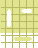Battleship: Sink your opponent's ships by answering questions correctly.# B3. Changing improper fractions to mixed numbers

Remember: To change an improper fraction to a whole or mixed number, divide the numerator by the denominator and place the remainder over the denominator |

Example; Convert 19/5 to a mixed number...

Solution; Divide 19 by 5 - When we divide 19 by 5 we find that 5 divides into 19 3 times with 4 left over. We can write this quotient as 3 with a remainder of 4, or as the mixed number 3 4/5.UsernamePasswordThis activity was created by a Quia Web subscriber. Learn more about QuiaCreate your own activities